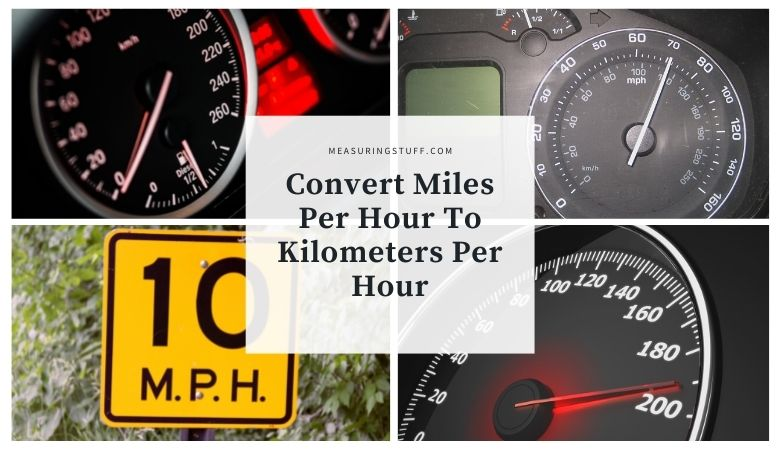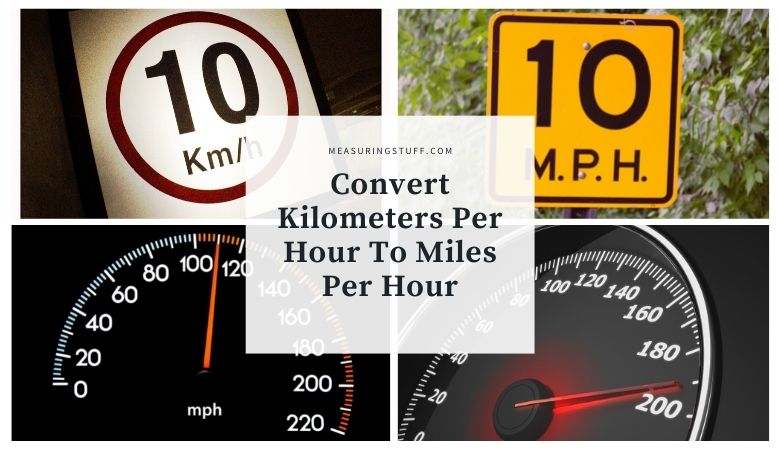# Speed## Convert Miles Per Hour To Kilometers Per Hour (MPH To KPH)

Similar to converting kilometers per hour into miles per hour, this can be done easily by using the following formula. There are 1.609344 kilometers per hour in 1 mile per hour. Simply multiply any miles per hour by 1.609344 to convert into kilometers per hour. For example, 1 MPH x 1.609344 = 1.609344 KPH. Another …## Convert Kilometers Per Hour To Miles Per Hour (KPH to MPH)

Converting kilometers per hour into miles per hour can be done easily by using the following formula. There are 0.62137119223733 miles per hour in 1 kilometer per hour. Simply multiply any kilometers per hour by 0.62137119223733 to convert into miles per hour. For example, 1 KPH x 0.62137119223733 = 0.62137119223733 MPH. Another example: Convert 120 …